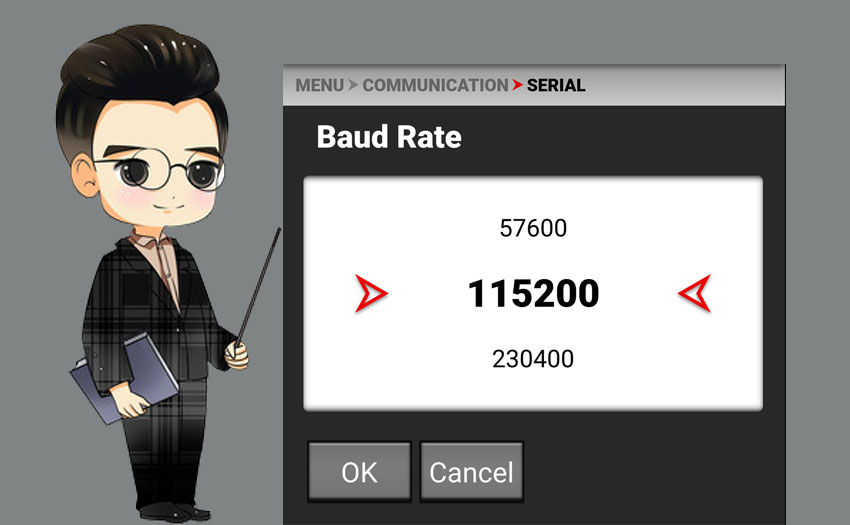# What's the baud Rate?The baud rate is the number of code symbols transmitted per second. It is a measure of the symbol transmission rate. It is expressed by the number of times and the carrier modulation state changes per unit time. 1 baud rate means transmission per second 1 symbol. the unit symbol is Bd. for electronic communication, the baud rate is the modulation rate, which refers to the rate at which the effective data signal modulates the carrier, that is, the number of times the carrier modulation state changes per unit time.Because of the extensive use of telex machines, the development of Modem has been promoted. In order to standardize and ensure effective communication, according to the physical characteristics of electricity and transmission media combined with the requirements of the use of serial devices, it is determined that the maximum transmission rate of the widely used RS232 can only be 115200, and then it is divided into two levels to obtain the common rate, such as 2400, 9600 , 19200, which is the baud rate. In order to adapt to these speeds, the concept of crystal frequency came into being. In addition, the reason why divide by two is used is that divide by 2 is the easiest in digital circuits.